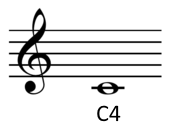# Convert pitch between Hz and music notation

Fill in one value and leave the other blank. Click the Convert button, and the missing value will be filled in.

 Cycles per second (Hz) Music notation (e.g. A5, F#2, Eb4, etc.)

If more than one box has a valid entry, the first one is used.

## Details

### Hertz

The frequency of a pitch in Hertz (Hz) is the number of cycles per second. For example, an A 440 has a pitch of 440 Hz, 440 vibrations per second.

### Musical notation

This page uses so-called scientific pitch notation. The C near the lower threshold of hearing is denoted C0. The C one octave higher is C1. The C two octaves above C0 is C2, etc. Middle C is C4.Notes besides C's take their octave number from the nearest C below. For example, the lowest note on a standard piano is A0, the A above C0. More details here.

In the form above use 'b' (the second letter of the English alphabet) for flat symbol ♭. So, for example, Eb4 is the E-flat above middle C.

If a frequency doesn't correspond exactly to a chromatic note, the difference is given in cents, where a cent is a hundredth of a semitone. For example, 440 Hz is A4 and 450 Hz is A4 + 39 cents.

More calculators like this one.Is there anything else I can help you with? Maybe something with signal processing?